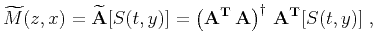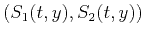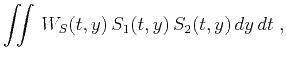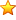Asymptotic pseudounitary stacking operatorsNext: ASYMPTOTIC PSEUDO-UNITARY OPERATOR PAIR Up: Asymptotic pseudounitary stacking operators Previous: ASYMPTOTIC INVERSION: RECONSTRUCTING THE

# LEAST-SQUARES INVERSION AND ADJOINT OPERATORS

Least-squares inversion is widely used in practice not only because it is applicable even when the asymptotic results are unavailable but also because of its ability to handle finite sampling effects that are difficult to handle in asymptotic theory (Ronen and Liner, 2000).

The theoretical least-squares inverse of operator (1) has the well-known form (Tarantola, 1987)(13)

wheredenotes pseudo-inverse, and the adjoint operatoris defined by the dot-product test:(14)

With a specified definition of the dot-product, the generalized inverse minimizes the following quantity, which is the squarednorm of the residual:(15)

In the case of integral operators, a natural definition of the dot-product is the double integral(16)(17)

The notion of the adjoint operator completely depends on the arbitrarily chosen definition of the dot product and norm in the model and data spaces. A simple way to change those definitions is to find some positive weightsin the model space andin the data space that define the dot products as follows:(18)(19)

To formally define the adjoint of a stacking operator, let us substitute the definition of the stacking operator (1) into the dot product (14), as follows:(20)

Assuming that the functionis monotone in, we can change the integration variabletoand rewrite equation (20) in the form(21)

wherehas the same meaning as in equation (8), and(22)

Comparing equations (21) and (14), we conclude that the adjoint operatoris defined by the equality(23)

Thus we have proven that the continuous adjoint of a stacking operator is another stacking operator. The adjoint operator has the same summation path as the asymptotic inverse (8), which guarantees the correct reconstruction of the kinematics of the input wavefield. The amplitude (weighting function) of the adjoint operator is directly proportional to the forward weighting according to equation (22). The coefficient of proportionality is the Jacobian of the transformation of the variablesand.

Similar results have been obtained for particular cases of stacking operators: velocity transform (Jedlicka, 1989; Thorson, 1984), Kirchhoff constant-velocity migration (Ji, 1994), and NMO (Crawley, 1995). In the appendix, I exemplify an application of least-squares inversion by reviewing inversion of the Radon operator and showing that it is precisely equivalent to the asymptotic result of the previous section.Asymptotic pseudounitary stacking operatorsNext: ASYMPTOTIC PSEUDO-UNITARY OPERATOR PAIR Up: Asymptotic pseudounitary stacking operators Previous: ASYMPTOTIC INVERSION: RECONSTRUCTING THE

2013-03-03